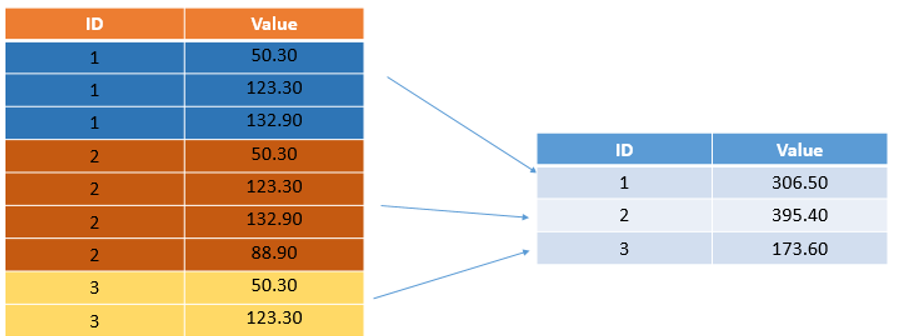# Python Pandas – GroupBy

Python Pandas – GroupBy: In this tutorial, we are going to learn about the Pandas GroupBy in Python with examples.
Submitted by Sapna Deraje Radhakrishna, on January 07, 2020

## Python Pandas – GroupBy

GroupBy method can be used to work on group rows of data together and call aggregate functions. It allows to group together rows based off of a column and perform an aggregate function on them.

Consider the below example, there are three partitions of IDS (1, 2, and 3) and several values for them. We can now group by the ID column and aggregate them using some sort of aggregate function. Here we are sum-ing the values and putting the values.### Groupby with Pandas

Create a dataframe from a dictionary

```import numpy as np
import pandas as pd

df = pd.DataFrame(data)
print(df)
```

Output

```     company     person  Sales
1  Microsoft  Nathaniel    123
2         FB    Sriansh    130
4         FB      Sarah    122
```

Following examples illustrate the 'GroupBy' function,

Example 1: GroupBy by 'company'

```# returns the groubBy object
print(df.groupby('company'))
'''
<pandas.core.groupby.generic.DataFrameGroupBy object at 0x7f1721585350>
'''

by_company = df.groupby('company')
#invoke aggregate function
print(by_company.mean())
'''
Sales
company
FB           126
Microsoft    123
'''
```

In the above example, we don't see the person column, because the data type is String and by no means, we can get mean of String variables, and hence Pandas automatically ignores any non-numeric values.

Below are some more examples of aggregate functions,

```print(by_company.sum())

'''
Output:
Sales
company
FB           252
Microsoft    123
'''
```
```print(by_company.std())

'''
Output:
Sales
company
FB          5.656854
Microsoft        NaN
'''
```

Note the return type of the values are by default a DataFrame, as illustrated below,

```std = by_company.std()
print(type(std))

'''
Output:
<class 'pandas.core.frame.DataFrame'>
'''
```

And, hence we can perform all the dataFrame functions such as,

```print(by_company.std().loc['FB'])

'''
Output:
Sales    5.656854
Name: FB, dtype: float64
'''
```

The above mentioned steps, all can be performed in a single step as follows,

```print(df.groupby('company').sum().loc['FB'])

'''
Output:
Sales    252
Name: FB, dtype: int64
'''
```

Some more aggregate functions are,

```print(df.groupby('company').count())

'''
Output:
person  Sales
company
FB              2      2
Microsoft       1      1
'''

print(df.groupby('company').max())

'''
Output:
person  Sales
company
FB           Sriansh    130
Microsoft  Nathaniel    123
'''

print(df.groupby('company').min())

'''
Output:
person  Sales
company
FB             Sarah    122
Microsoft  Nathaniel    123
'''
```

### Using GroupBy with describe method

The describe() method returns a bunch of useful information all at once.

```print(df.groupby('company').describe())

'''
Output:
Sales                    ...
count   mean        std  ...    50%    75%    max
company                            ...
FB          2.0  126.0   5.656854  ...  126.0  128.0  130.0
Google      2.0  172.0  39.597980  ...  172.0  186.0  200.0
Microsoft   1.0  123.0        NaN  ...  123.0  123.0  123.0

[3 rows x 8 columns]
'''
```

The format of the description can be changed using transpose() method,

```print(df.groupby('company').describe().transpose())

'''
Output:
Sales count    2.000000    2.00000        1.0
mean   126.000000  172.00000      123.0
std      5.656854   39.59798        NaN
min    122.000000  144.00000      123.0
25%    124.000000  158.00000      123.0
50%    126.000000  172.00000      123.0
75%    128.000000  186.00000      123.0
max    130.000000  200.00000      123.0
'''
```

Preparation